## LVEDV Z-Scores

The second of two recent updates to app.parameterz.com is the addition of the left ventricular volume and dimension data supplied by this recent article:

Normalized end-systolic volume and pre-load reserve predict ventricular dysfunction following surgery for aortic regurgitation independent of body size.
Gentles TL, French JK, Zeng I, Milsom PF, Finucane AK, Wilson NJ.
JACC Cardiovasc Imaging. 2012 Jun;5(6):626-33.
(The equations are provided via an online supplement, supplied to me by the authors.)
Although the article describes equations for LV function and dimensions, I use only the dimensional z-scores. The form of these equations is that of an allometric relationship with BSA, with log-log transformation of both the measured value and the BSA.
The formula used for estimating BSA is not described within the article/supplement; I am awaiting a response from the authors on this. In the meantime, calculations are made using the Haycock formula.

### LVEDD and LVESD

The allometric equations for the LVEDD and LVESD use exponents of around 0.4, which is slightly less than what would be expected by the theory of geometric similarity: 0.5. Numerous other authors have discovered linear relationships when correcting/adjusting linear dimensions (cm) to body surface area (m2). Additionally, the LVESD values seem a bit off when compared to other available sources: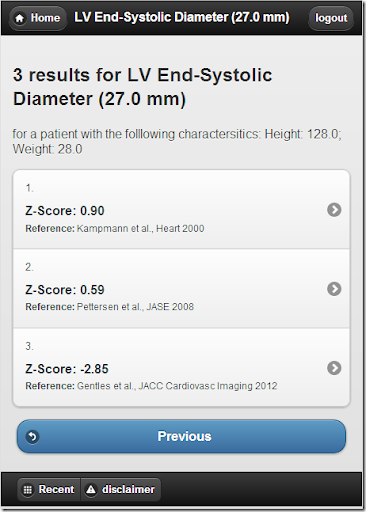(I have contacted the authors; awaiting their response)

### LVEDV and LVESV

Data for the left ventricular volumes is also in the form of an allometric relationship with BSA, with an exponent of around 1.1. Again, this differs somewhat from what would be predicted by geometric similarity, and by other empirical evidence. The closer the exponent is to 1.0, the more the relationship is strictly linear with BSA, which is unexpected, particularly since the authors recognize this peril:
However, the relationship between body size and LV size is nonlinear
Yet, when you plot out the predicted values, it looks quite linear: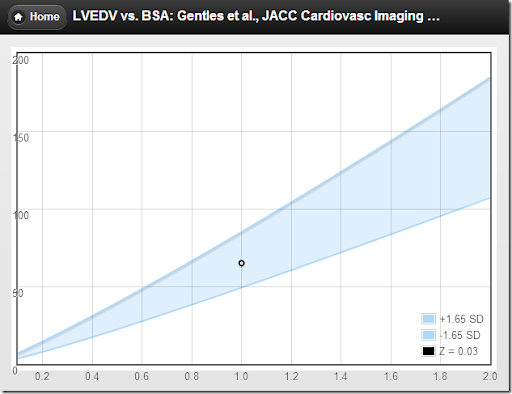versus an allometric equation with an exponent of 1.38 (Lytrivi et al.):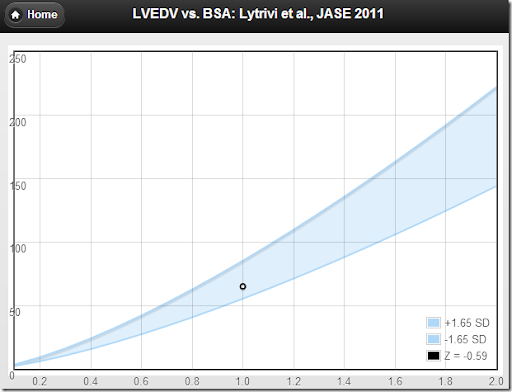Anyhow, given the paucity of ventricular volume z-score equations, these are now included among other pediatric echo z-score calculations at

### app.parameterz.com

***update Dec 2012***
The author has advised me that there was an error with the online supplement.
"The intercept for ESD should be 2.56 NOT 3.56 as is on the web"
The app now uses the updated value.

## Left Atrial Volume Z-Scores

Calculating BSA-adjusted z-scores of LA volume using an allometric equation
The first of two new calculations added to app.parameterz.com is based this recent article:

Normal values of left atrial volume in pediatric age group using a validated allometric model.
Bhatla P, Nielsen JC, Ko HH, Doucette J, Lytrivi ID, Srivastava S.
Circ Cardiovasc Imaging. 2012 Nov 1;5(6):791-6.

Similar to how this same group calculates z-scores for LV end-diastolic volume, they found an allometric equation that scales well with body size (in this case, two equations) and they index to that. For left atrial volume, there is one equation for BSA ≤ 1.0 and another equation for BSA > 1.0. The allometric exponent for the bigger kids, i.e., BSA1.08, is not much different than just indexing to BSA, which strikes me as interesting.
I think the stratification into two groups would be more palatable if the interface was seamless, but in this case it is not. Let’s have a look at two patients with an LA volume of 34ml, one just under 1.0 meters squared and the other, just above.
And their accompanying plots:

#### BSA = 0.96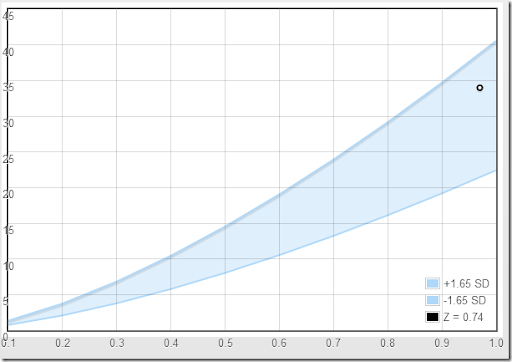#### BSA = 1.03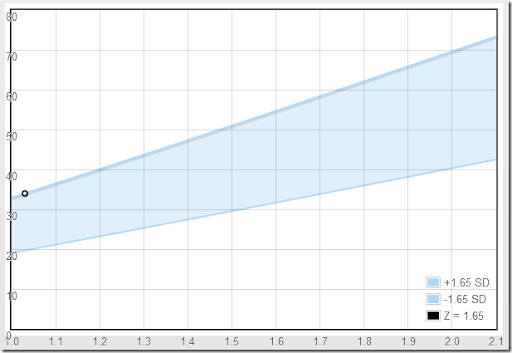For the smaller patient, the LA volume of 34 ml is well within the normal range, but for the slightly larger patient, the same LA volume is now at the upper limit of normal. Weird, huh?
Plus, it gets a little more interesting. If we assume that 125-131 cm is about the appropriate height for a 7 year old, and we plug the same data into another calculation, the indexed left atrial volume is definitely large, with a z-score of 3.5: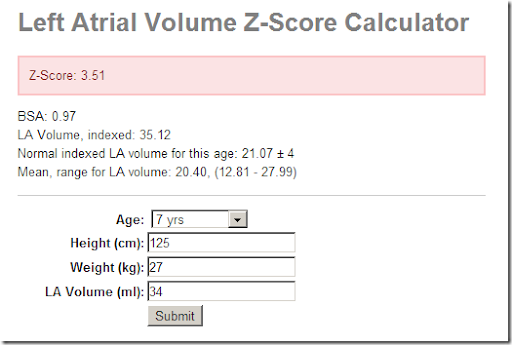Anyhow (as always) I don’t pretend to have the answers— I just pose questions. Feel free to play around with this and other z-score calculations at: